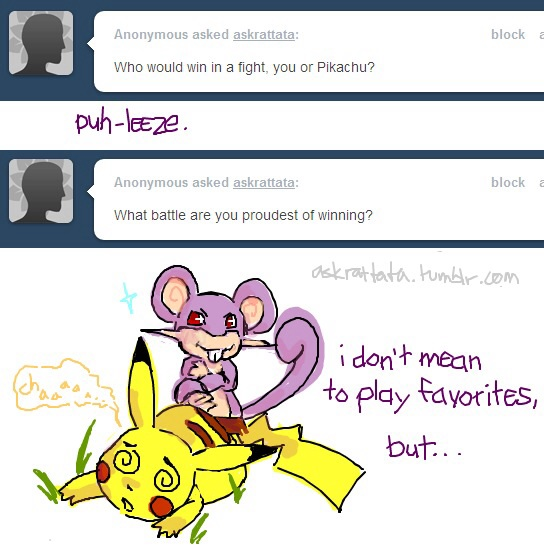# Themed Challenge 2 (Manga/Anime): The Exhaustive Battle

Probability Level 4Pikachu and a wild Rattata have been battling for a long time. They have been fighting for so long that their accuracy is haywire, and their health is one on-target hit away from being a K.O. Any attack now, however powerful or weak, if on target, could defeat the opponent.

At this stage of battle, Rattata has $2$ attacks. He can use Tackle, which has a $\frac{1}{3}$ chance of being on target. He can also use Hyper Fang, but only after $2$ moves have occurred (i.e. Rattata moves, then Pikachu moves, then Rattata can use it or save for a later move). This attack has a $\frac{2}{3}$ accuracy rate.

Pikachu, on the other hand has $3$ attacks. One is Quick Attack, which has a $\frac{1}{3}$ probability of being on target. Another attack is ThunderShock, which has a $\frac{1}{2}$ probability of being on target, but can only be used after $2$ moves. Finally, Pikachu can use ShockWave, a move with Infinite Accuracy, which, mathematically speaking has an accuracy rate of $1$. However, it can only be used after $4$ moves.

In this battle, the highest probability of Rattata winning and defeating Pikachu is given by $\frac{M}{N}$ , where $M,N$ are co-prime integers. Find $M+N$.

×